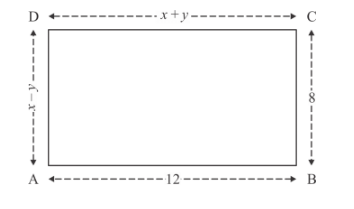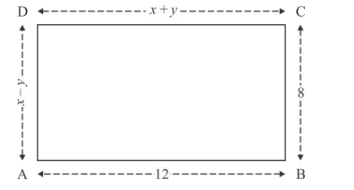# In Fig. 4, ABCD is a rectangle. Find the values of x and y.`
Question:

In Fig. 4, ABCD is a rectangle. Find the values of x and y.Solution:

The following diagram is givenThe given diagram is rectangle and we know that in rectangle the face to face sides are same. Therefore

$x+y=12 \ldots \ldots(1)$

$x-y=8 \ldots \ldots$ (2)

Now we are adding the equations (1) and (2) to have

$2 x=20$

$\Rightarrow x=10$

Put the value of in equation (1), we get

$10+y=12$

$\Rightarrow y=12-10$

$\Rightarrow y=2$

Hence, = 10 and = 2

Therefore the values of $x$ and $y$ are $x=10, y=2$